Characterizations of Certain Doubly Truncated Distribution Based on Order Statistics | OMICS International
Journal of Applied & Computational Mathematics

# Characterizations of Certain Doubly Truncated Distribution Based on Order Statistics

1Department of Statistics, Faculty of Science, King Abdulaziz University, Saudi Arabia

2Department of Statistics, Faculty of Science for Girls, King Abdulaziz University, Saudi Arabia

*Corresponding Author:
Shawky AI
Department of Statistics, Faculty of Science
King Abdulaziz University, P.O. Box 80203
Jeddah 21589, Saudi Arabia
Tel: +966 800 116 9528
E-mail: [email protected]

Received date: October 24 , 2016; Accepted date: November 23, 2016; Published date: November 29, 2016

Citation: Shawky AI, Badr MM (2016) Characterizations of Certain Doubly Truncated Distribution Based on Order Statistics. J Appl Computat Math 5:329. doi: 10.4172/2168-9679.1000329

Copyright: © 2016 Shawky AI, et al. This is an open-access article distributed under the terms of the Creative Commons Attribution License, which permits unrestricted use, distribution, and reproduction in any medium, provided the original author and source are credited.

Visit for more related articles at Journal of Applied & Computational Mathematics

#### Abstract

In this paper, we characterize doubly truncated classes of absolutely continuous distributions by considering the conditional expectation of functions of order statistics. Specific distributions considered as a particular case of the general class of distributions are Weibull, Pareto, Power function, Rayleigh and Inverse Weibull.

#### Keywords

Double truncated; Order statistics; Conditional expectation; Weibull, Pareto; Rayleigh; Inverse Weibull distributions

AMS 2000 Subject Classification: 62E10; 62G30

#### Introduction

The order statistics arise naturally in many real life applications and it is considered as an increasingly important subject. Articles relating to this area have appeared in numerous different publications. Many authors have studied order statistics; for example, David , Balakrishnan and Cohen , Arnold et al. , David , David and Nagaraja  and Mahmoud et al. [6,7]. Several authors discussed conditional expectations, for example, Balakrishnan and Sultan , Mohie El-Din et al. , Abu-Youssef , Abd- El-Mougod , Shawky and Abu-Zinadah , Shawky and Bakoban  and Pushkarna et al. .

Let X1:n ≤ X2:n ≤ …≤ Xn:n be the first n order statistics based on distribution with probability density function (pdf) f(x) and cumulative distribution function (cdf) F(x). Then the pdf of the rth order statistics, Xr:n, 1 ≤ r ≤ n, is given by (see David (1981))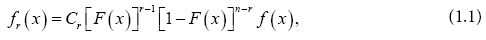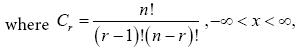and the joint pdf of two order statistics Xr:n and Xs:n, 1 ≤ r ≤ s ≤ n is given by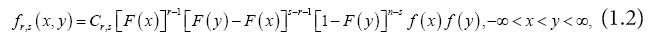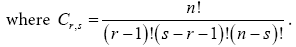The doubly truncated case of a distribution is the most general case since it includes the right truncated, left truncated and non-truncated distributions as special cases, Joshi , Balakrishnan and Joshi , Khan and Ali  and Ahmad , among others, investigated doubly truncated distributions.

Suppose that the random variable X has a cdf F(x) and pdf f(x), where α ≤ x ß. Let, for given ε and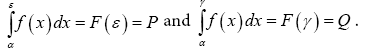Then the doubly truncated pdf of X, say g(x), and cdf, say G(x), are given respectively by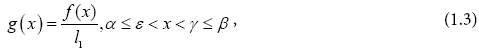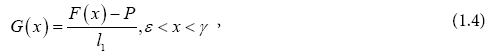where

l1=Q – P, G(ε)=0 and G(γ)=1.

The conditional density function of Xs:n=y, given that Xr:n=x is given  by

Also, the conditional density function of Xr:n=x, given that Xs:n=y is given by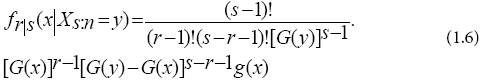Let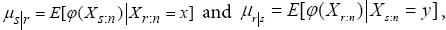where ? (.) is a monotonic, continuous and differentiable function on the interval (α,ß). For abbreviation, we will denote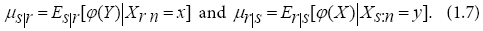#### Main Results

In this section, we characterize three general classes of distributions,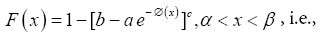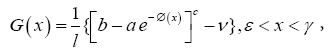where

v=1–P, l=P – Q, P=F(ε), Q=F(γ), G(ε)=0, G(γ)=1,

F(α)=0, F(ß)=1, and a ≠ 0, c 0, b are finite constants.

F(x)=1 – [a – b ∅(x)]c, α < x < ß, i.e.,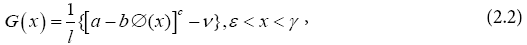where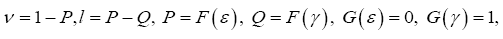F(α)=0, F(ß)=1, and b ≠ 0, c ≠ 0, a are finite constants.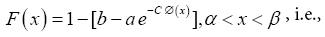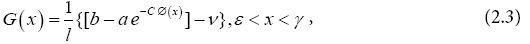where

v=1 – P, l=P – Q, P=F(ε), Q=F(γ), G(ε)=0, G(γ)=1,

F(α)=0, F(ß)=1, and C ≠ 0, a > 0, b > 0 are finite constants.

Note: If we put l=–1, v=1, thus G(x) reduces to complete cdf of x, i.e. F(x), α < x < ß.

Let X be an absolutely continuous random variables with pdf g(x), cdf G(x) and ∅(x) is a monotonic, continuous and differentiable function on (ε,γ).

Theorems 1-4 given below characterize the general class given by (2.1), Theorems 5-8 characterize the general class given by (2.2), while Theorems 9-12 characterize the general class given by (2.3).

#### Theorem 1

Referring to (1.6), (1.7) and (2.1), then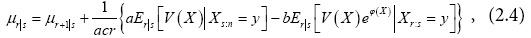where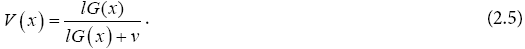Proof

It is clear from (1.6) and (1.7) that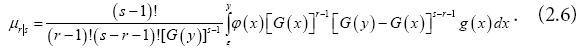Integrating (2.6) by parts, we get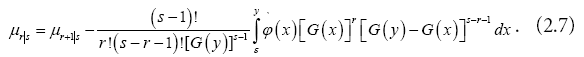Differentiating (2.1) with respect to x, we have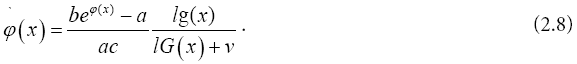From (2.7) and (2.8), we obtain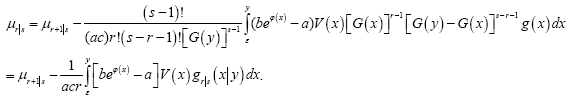(2.9)

Simplifying (2.9), we get (2.4). Thus, the theorem is proved.

#### Theorem 2

Referring to (1.6), (1.7), then (2.1) if and only if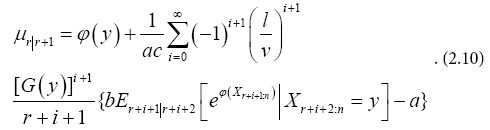Proof

It is clear that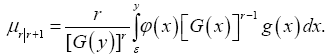Integrating by parts, we get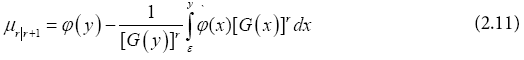Compensation for (2.8) in (2.11), we have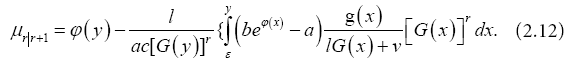Expand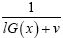and compensation for (2.12), after some simplification, we get (2.10). Thus (2.1) implies (2.10). Now from (1.6) and (2.10), we obtain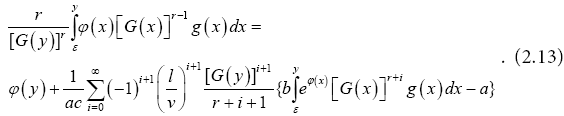Taking the derivative, we get

which gives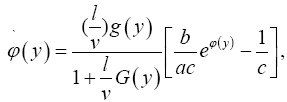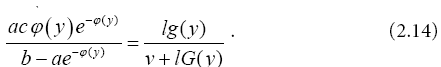Integrate (2.14), hence G(y) has the form (2.1), and so (2.10) implies (2.1).

Special case: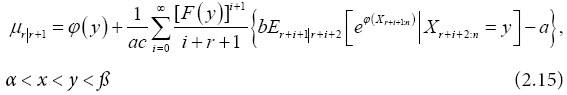the relation (2.15) is before doubly truncated case.

#### Theorem 3

Referring to (1.5), (1.7) and (2.1), then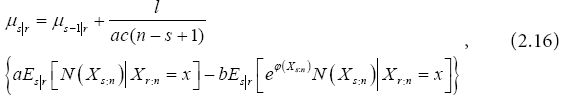where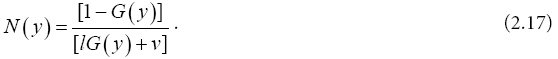It is clear from (1.5) and (1.7) that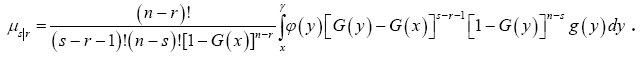Integrating by parts, we get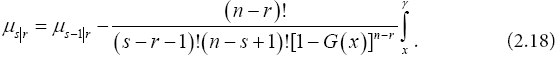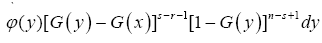Substituting (2.7) in (2.18), we get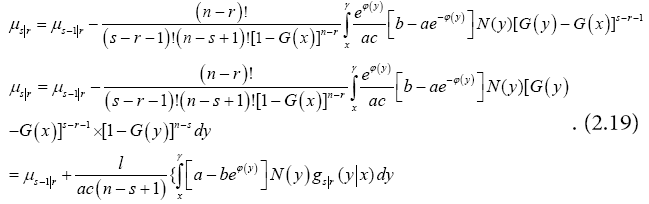After some simplification, we get (2.16).

#### Theorem 4

Referring to (1.5), (1.7), then (2.1) if and only if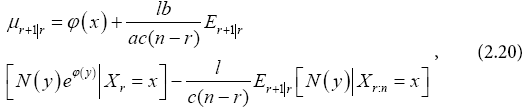where N(y) is defined in (2.17).

Proof

It is clear that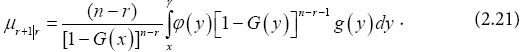Integrating by parts, we get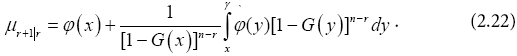Compensation for (2.8) in (2.22), we have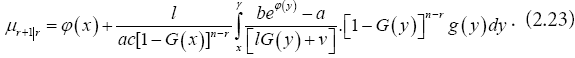Simplifying (2.23), we obtain (2.20). Thus (2.1) implies (2.20), i.e. the necessary condition is proved. To prove the sufficient condition, from (2.20) and (1.7), we have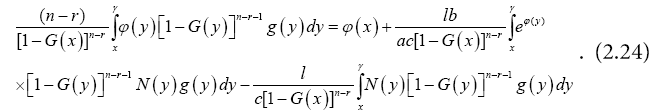Taking the derivative of (2.24) with respect to x, we get (2.8), and integrate it we have (2.1), thus (2.20) implies (2.1). Then, the Theorem is proved.

Special case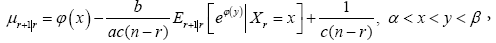it is before doubly truncated case (Table 1).

Name [lG(x)+ν] φ(x) (l,ν)  (a,b,c)
Weibull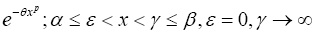xp
qxp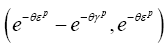(–1,0,θ)
(–1,0,1)
Pareto θp x-p; αε < x < γβ, ε = 0, γ ln(x)?
ln[xp]
(θp(γp–ε–p), θp ε–p) (–θ,0,p)
(–θp,0,–1)
Power function 1–θ–p xp,α ε < x < γ β, ε = 0, γ    ∞   (θpp–γp), 1–θp εp) (1,1,1)
(θp,1,1)
Rayleigh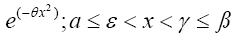x2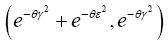(–1,0,θ)
Inverse Weibull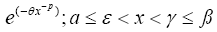θxp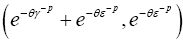(–1,0,1)

Table 1: Example of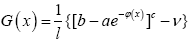distributions.

#### Theorem 5

Referring to (1.6), (1.7) and (2.2), then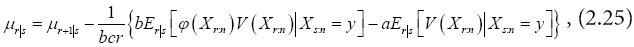where V(x) is defined in (2.5).

Proof

As before in Theorem (1), differentiate (2.2) with respect to x, we have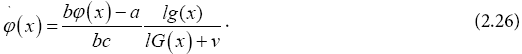Compensation for (2.26) in (2.7), we get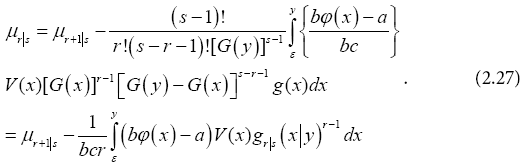Simplifying (2.27), we obtain (2.25). Thus, the Theorem is proved.

#### Theorem 6

Referring to (1.6), (1.7), then (2.2) if and only if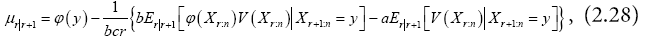Where V(x) is defined in (2.5).

Proof

As before in Theorem (2), from (2.26) and (2.11), we have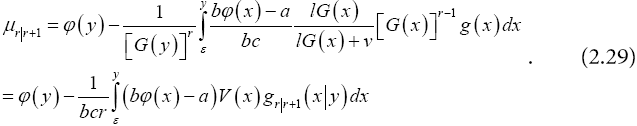Therefore, we get (2.28), then (2.2) implies (2.28). To prove the sufficient condition, from (2.28) and (1.7), we obtain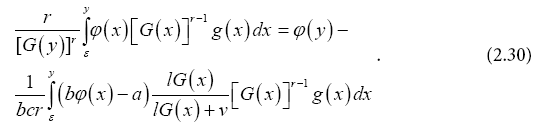Taking the derivative, we get (2.26) and we obtain, after integration, (2.2). Thus (2.28) implies (2.2).

Special case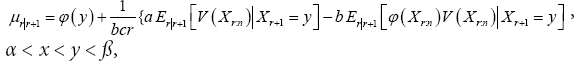it is before doubly truncated case.

#### Theorem 7

Referring to (1.5), (1.7) and (2.2), then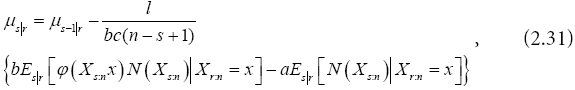where N(y) is defined in (2.17).

Proof

As before in Theorem (3), compensation for (2.26) in (2.18), we have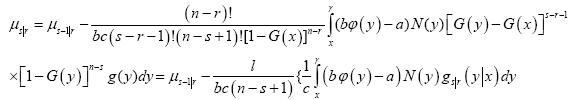After simplification, we get (2.31). Then (2.2) implies (2.31).

#### Theorem 8

Referring to (1.5), (1.7), then (2.2) if and only if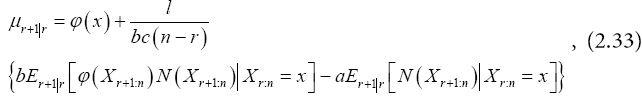where N(y) is defined in (2.17).

Proof

As before in Theorem (4), compensation for (2.26) in (2.23), we have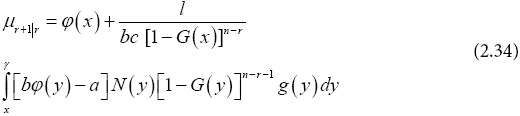Then, we obtain (2.35). Thus (2.2) implies (2.33). Now from (1.7) and (2.33) we get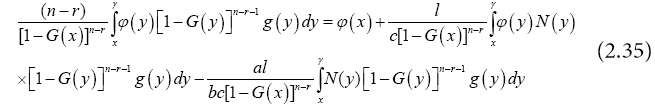Taking the derivative with respect to x, we obtain (2.26), and integrate it we have (2.2), thus (2.33) implies (2.2).

Hence, the Theorem is proved. Special caseReturn to (2.33), then put l=–1, v=1, we get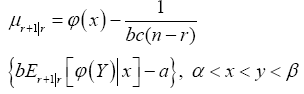it is before doubly truncated case (Table 2).

Name [lG(x) + v] j(x) (l,v) (a,b,c)
Weibull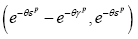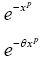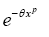(-1,0,θ)
Power function 1– θp xp,
α ε < x < γβ, ε = 0, γ → ∞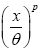xp
(θp (εp + γp), 1–θpεp) (1,1,1)
(q–p,1,1)
Rayleigh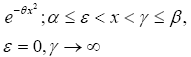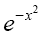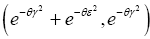(–1,0,θ)
Inverse Weibull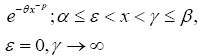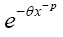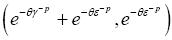(0,–1,1)

Table 2: Example of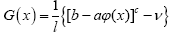distributions.

#### Theorem 9

Referring to (1.6), (1.7) and (2.3), then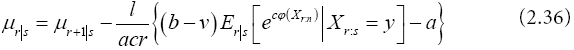Proof

As given in Theorems (1) and (5), , differentiate (2.3) with respect to x, we have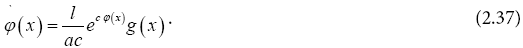Compensation for (2.37) in (2.7), we get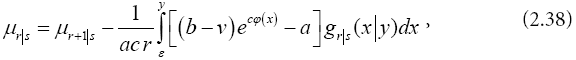which gives (2.36). Thus, (2.3) implies (2.36).

#### Theorem 10

Referring to (1.6) and (1.7), then (2.3) if and only if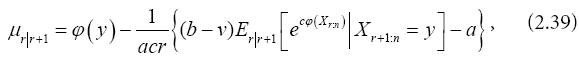Proof

As given previously of Theorems (2) and (6), substituting from (2.26) in (2.11), we have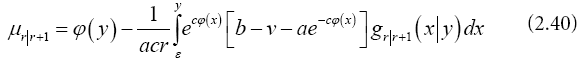After some simplification, we get (2.36). Then (2.3) implies (2.36). Now from (1.7) and (2.36), we obtain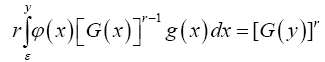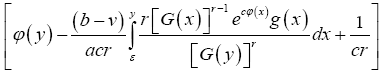Taking the derivative with respect to y, we get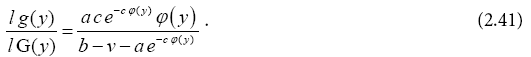Integrate (2.41), we obtain (2.3).

Special case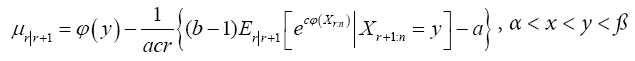it is before doubly truncated case.

#### Theorem 11

Referring to (1.5), (1.7) and (2.3), then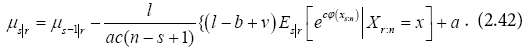Proof

As previously in Theorems (3) and (7), from (2.37) in (2.18), we have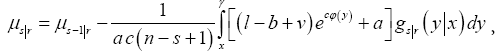which gives (2.42).

#### Theorem 12

Referring to (1.5) and (1.7), then (2.3) if and only ifProof

Similarly as given in Theorems (4) and (8), we easily prove it.

Special case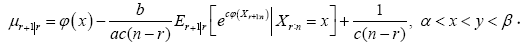The relation before doubly truncated case (Table 3).

Name [lG(x) + v] j(x) (l,v) (a,b,c)
Power distribution 1–θp xp,α ε < x < γ β, ε = 0, γ = θ Ln[1θpxp] (θp(εp+ γp), 1θ–pεp) (1,0,–1)
Weibull eθxp, αε < x < γβ, ε = 0, γ → ∞ θxp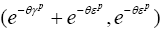(–1,0,1)
Burr (1+θxp)γ, αε < x < γβ, ε = 0, γ→∞ ln[1 + qxp] (θγp) + θεp +1), 1+θεp) (–1,0,γ)
Inverse Weibull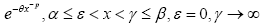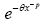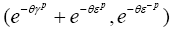(–1,0,1)

Table 3: Example of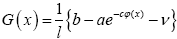distributions.

#### Conclusion

It was obtained recurrence relations based on order statistics without truncated and doubly truncated, and have been getting function of various distributions new by using certain parameters.

#### Acknowledgement

This paper was founded by the Deanship of Scientific Research (DSR), King Abdulaziz University,Jeddah, under grant No. (65-363-D1431).The authors, therefore, acknowledge with thanks DSR technical and financial support.

#### References

Select your language of interest to view the total content in your interested language

### Article Usage

• Total views: 9889
• [From(publication date):
October-2016 - Dec 08, 2019]
• Breakdown by view type
• HTML page views : 9762Can't read the image? click here to refresh